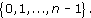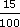• 1.   The condition ‘is equal to’ is a relation on any set of real numbers because, for any x, y in the set, the statement ‘x is equal to y’ is either definitely true or definitely false. This relation is usually denoted by the symbol =. For this relation, each real number in the set is related only to itself!

• 2.   The condition ‘is less than’ is a relation on any set of real numbers, and we usually
Author(s): The Open University

The Division Algorithm tells us that all the possible remainders on division by an integer n lie in the setWe denote this set by [Image_Link]https://www.open.edu
Author(s):
The Open University

We will now discuss complex numbers and their properties. We will show how they can be represented as points in the plane and state the Fundamental Theorem of Algebra: that any polynomial equation with complex coefficients has a solution which is a complex number. We will also define the function exp of a complex variable.

Earlier we mentioned several sets of numbers, including [Image_Link]https://www.open.edu/openlearn/ocw/pluginfile.php/89651/mod_ouc
Author(s):
The Open UniversityStudy another free course

There are more than 800 courses on OpenLearn for you to
Author(s): The Open University

An important idea when calculating volumes of simple shapes is that of a cross-section. In the case of the rectangular box considered above, it is possible to slice through the box horizontally so that the sliced area is exactly the same as the area of the base or top; in other words, the areas of the horizontal cross-sections are equal.

[Image_Link]https://www.open.edu/openlearn/ocw/pluginfile.php/94675/mod_oucontent/ouco
Author(s): The Open University

What is a volume? The word usually refers to the amount of three-dimensional space that an object occupies. It is commonly measured in cubic centimetres (cm3) or cubic metres (m3).

A closely related idea is capacity; this is used to specify the volume of liquid or gas that a container can actually hold. You might refer to the volume of a brick and the capacity of a jug – but not vice versa. Note that a container with a particular volume will not nec
Author(s): The Open University

Question 1

Find the area of a circle of (a) radius 8 cm, and (b) radius 15 m.

• (a)

Author(s): The Open University

Question 1

Find the area of each of these shapes.

[Image_Link]https://www.open.edu/openlearn/ocw/pluginfile.php/94675/mod_oucontent/oucontent/779/39f826a0
Author(s): The Open University

In this section we shall define the complex number system as the set R × R (the Cartesian product of the set of reals, R, with itself) with suitable addition and multiplication operations. We shall define the real and imaginary parts of a complex number and compare the properties of the complex number system with those of the real number system, particularly from the point of view of analysis.

Author(s): The Open University

Section 6 contains solutions to the exercises that appear throughout sections 1-5.

Click the link below to open the solutions (13 pages, 232KB).

Section 6

Author(s): The Open University

In Section 5 we show how functions may be used to sketch curves in the plane, even when these curves are not necessarily the graphs of functions.

Click the link below to open Section 5 (8 pages, 151KB).

Section 5

Author(s): The Open University

Discount can be calculated in the same way as an increase by a percentage. For example, £8 with 15% discount means you actually pay

£8 less (15% of £8)

15% of 8 =× 8 =
Author(s): The Open University

Fractions and decimals can also be converted to percentages, by multiplying by 100%.

So, for example, 0.17, 0.3 andcan be expressed as percentages as follows:

0.17 × 100% = 17%;

<
Author(s): The Open University

Activity 3

A local supermarket sells a popular breakfast cereal in a ‘Large Pack’ and ‘New Extra Large Pack’. They are both being sold at ‘knock down’ prices. The large pack contains 450 g of cereal priced at �
Author(s): The Open University

Ratios crop up often in official statistics. The government wants the teacher–pupil ratio in schools to be increased to one teacher to thirty pupils or less. The birth rate has fallen: the ratio of children to women of child bearing age has gone down. It used to be 2.4 to 1, and now it is 1.9 to 1. Predictions for the ratio of working adults to retired adults is disturbing. Predictions are, that by 2030 the ratio will be two working adults to every retired person, instead of three to one no
Author(s): The Open University

The content acknowledged below is Proprietary (see and conditions made available under a Creative Commons Attribution-NonCommercial-ShareAlike 4.0 Licence) and used under licence.

Course image: rod
Author(s): The Open University

Mathematical processes are different from content in that they overarch the subject and are not thought of as hierarchical. A list of processes could contain:

• problem-solving (including investigating);

• mathematical modelling;

• reasoning;

• communicating;

• making connections (including applying mathematics); and

• using tools.

Each of the six processes listed here repre
Author(s): The Open University

World in transition: Managing Resources
Do you take your access to water for granted? The Peruvian and Tanzanian communities featured in this album certainly don’t. This album examines how development agencies can empower communities to help themselves by introducing simple technologies, and facilitate the sharing of ideas through education. In the Andean mountains, scarce supplies of water and agricultural challenges give rise to conflict; but the changes engineered by development agencies can start to show a way out of poverty. Me
Author(s): The OpenLearn team

Understanding the environment: Learning and communication
There is increasing recognition that the reductionist mindset that is currently dominating society, rooted in unlimited economic growth unperceptive to its social and environmental impact, cannot resolve the converging environmental, social and economic crises we now face. The primary aim of this free course, Understanding the environment: Learning and communication, is to encourage the shift away from reductionist and human centred thinking towards a holistic and ecological worldview.
Author(s): Creator not set

Introducing the environment: Ecology and ecosystems
What is ecology and why is it important to our understanding of the world around us? This free course, Introducing the environment: Ecology and ecosystems, looks at how we can study ecosystems to explore the effect that humans are having on the environment. First published on Fri, 18 Mar 2016 as Author(s): Creator not set